# A note on the polynomial-exponential Diophantine equation (an − 1)(bn − 1) = x2

Yasutsugu Fujita and Maohua Le
Notes on Number Theory and Discrete Mathematics
Print ISSN 1310–5132, Online ISSN 2367–8275
Volume 27, 2021, Number 3, Pages 123–129
DOI: 10.7546/nntdm.2021.27.3.123-129

## Details

### Authors and affiliations

Yasutsugu FujitaDepartment of Mathematics, College of Industrial Technology, Nihon University
2-11-1 Shin-ei, Narashino, Chiba, Japan

Maohua LeInstitute of Mathematics, Lingnan Normal College
Zhangjiang, Guangdong, 524048 China

### Abstract

For any positive integer, let ord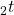denote the order of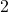in the factorization of. Let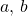be two distinct fixed positive integers with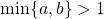. In this paper, using some elementary number theory methods, the existence of positive integer solutions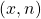of the polynomial-exponential Diophantine equation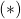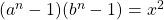with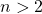is discussed. We prove that if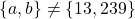and ord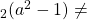ord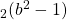, thenhas no solutionswith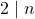. Thus it can be seen that if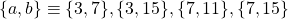or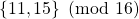, where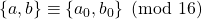means either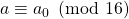and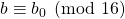or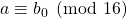and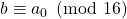, thenhas no solutions.

### Keywords

• Polynomial-exponential Diophantine equation
• Pell’s equation

• 11D61

### References

1. Bennett, M. A., & Skinner, C. M. (2004). Ternary Diophantine equations via Galois representations and modular forms. Canadian Journal of Mathematics, 56, 23–54.
2. Cohn, J. H. E. (2002). The Diophantine equation (an − 1)(bn − 1) = x2. Periodica Mathematica Hungarica, 44, 169–175.
3. Guo, X.-Y. (2013). A note on the Diophantine equation (an − 1)(bn − 1) = x2. Periodica Mathematica Hungarica, 66, 87–93.
4. Hajdu, L., & Szalay, L. (2000). On the Diophantine equations (2n − 1)(6n − 1) = x2 and (an − 1)(akn − 1) = x2. Periodica Mathematica Hungarica, 40, 141–145.
5. Ishii, K. (2016). On the exponential Diophantine equation (an − 1)(bn − 1) = x2. Publicationes Mathematicae Debrecen, 89, 253–256.
6. Le, M.-H. (2009). A note on the exponential Diophantine equation (2n − 1)(bn − 1) = x2. Publicationes Mathematicae Debrecen, 74, 401–403.
7. Le, M.-H., & Soydan, G. (2020). A brief survey on the generalized Lebesgue–Ramanujan–Nagell equation. Surveys in Mathematics and its Applications, 15, 473–523.
8. Li, L., & Szalay, L. (2010). On the exponential Diophantine equation (an − 1)(bn − 1) = x2. Publicationes Mathematicae Debrecen, 77, 465–470.
9. Li, Z.-J. (2011). Research for the solution of the Diophantine equation (an − 1)(bn − 1) = x2. Master’s thesis, Wuhu: Anhui Normal University (in Chinese).
10. Li, Z.-J., & Tang, M. (2010). On the Diophantine equation (2n − 1)(an − 1) = x2. Journal of Anhui Normal University, Natural Science, 33, 515–517 (in Chinese).
11. Li, Z.-J., & Tang, M. (2011). A remark on a paper of Luca and Walsh. Integers, 11, 827–832.
12. Liang, M. (2012). On the Diophantine equation (an − 1)((a + 1)n − 1) = x2. Journal of Mathematics (Wuhan), 32, 511–514 (in Chinese).
13. Luca, F., & Walsh, P. G. (2002). The product of like-indexed terms in binary recurrences. Journal of Number Theory, 96, 152–173.
14. Mordell, L. J. (1969). Diophantine Equations, London: Academic Press.
15. Noubissie, A., Togbe, A., & Zhang, Z.-F. (2020). On the exponential Diophantine equation (an − 1)(bn − 1) = x2. The Bulletin of the Belgian Mathematical Society, 27, 161–166.
16. Szalay, L. (2000). On the Diophantine equation (2n − 1)(3n − 1) = x2. Publicationes Mathematicae Debrecen, 57, 1–9.
17. Tang, M. (2011). A note on the exponential Diophantine equation (am − 1)(bn − 1) = x2. Journal of Mathematical Research and Exposition, 31, 1064–1066.
18. Yang, H., Pei, Y.-T., & Fu, R.-Q. (2016). The solvability of the Diophantine equation (an − 1)((a + 1)n − 1) = x2. Journal of Xiamen University, Natural Science, 55, 91–93 (in Chinese).
19. Yang, S.-C., Wu, W.-Q., & Zheng, H. (2011). The solution of the Diophantine equation (an − 1)(bn − 1) = x2. Journal of Southwest University, Natural Science, 37, 31–34 (in Chinese).
20. Yuan, P.-Z., & Zhang, Z.-F. (2012). On the Diophantine equation (an − 1)(bn − 1) = x2. Publicationes Mathematicae Debrecen, 80, 327–331.

## Cite this paper

Fujita, Y. & Le, M. (2021). A note on the polynomial-exponential Diophantine equation (an − 1)(bn − 1) = x2. Notes on Number Theory and Discrete Mathematics, 27(3), 123-129, doi: 10.7546/nntdm.2021.27.3.123-129.